# Hermitian form

(diff) ← Older revision | Latest revision (diff) | Newer revision → (diff)

on a left-moduleA mappingthat is linear in the first argument and satisfies the conditionHereis a ring with a unit element and equipped with an involutory anti-automorphism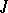. In particular,is a sesquilinear form on. The moduleitself is then called a Hermitian space. By analogy with what is done for bilinear forms, equivalence is defined for Hermitian forms (in another terminology, isometry) and, correspondingly, isomorphism (isometry) of Hermitian spaces (in particular, automorphism). All automorphisms of a Hermitian formform a group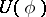, which is called the unitary group associated with the Hermitian form; its structure has been well studied whenis a skew-field (see Unitary group).

A Hermitian form is a special case of an-Hermitian form (whereis an element in the centre of), that is, a sesquilinear formonfor whichWhen, an-Hermitian form is Hermitian, and whenthe form is called skew-Hermitian or anti-Hermitian. If, a Hermitian form is a symmetric bilinear form, and a skew-Hermitian form is a skew-symmetric or anti-symmetric bilinear form. If the mapping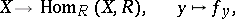wherefor any, is bijective, thenis called a non-degenerate Hermitian form or a Hermitian scalar product on.

Ifis a free-module with a basis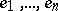, then the matrix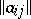, where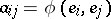, is called the matrix ofin the given basis; it is a Hermitian matrix (that is,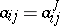). A Hermitian formis non-degenerate if and only ifis invertible. Ifis a skew-field, if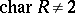, and ifis finite-dimensional over, thenhas an orthogonal basis relative to(in which the matrix is diagonal).

Ifis a commutative ring with identity, if, and if the matrix ofis definite, then its determinant lies in. Under a change of basis inthis determinant is multiplied by a non-zero element ofof the form, whereis an invertible element of. The determinant regarded up to multiplication by such elements is called the determinant of the Hermitian form or of the Hermitian space; it is an important invariant and is used in the classification of Hermitian forms.

Letbe commutative. Then a Hermitian formongives rise to a quadratic form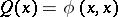onover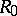. The analysis of such forms lies at the basis of the construction of the Witt group ofwith an involution (see Witt ring; Witt decomposition; Witt theorem). Whenis a maximal ordered field, then the law of inertia extends to Hermitian forms (and there arise the corresponding concepts of the signature, the index of inertia, and positive and negative definiteness). Ifis a field and, thenis a quadratic Galois extension of, and isometry of two non-degenerate Hermitian forms overis equivalent to isometry of the quadratic forms overgenerated by them; this reduces the classification of non-degenerate Hermitian forms overto that of non-degenerate quadratic forms over.

If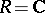andis the involution of complex conjugation, then a complete system of invariants of Hermitian forms over a finite-dimensional space is given by the rank and the signature of the corresponding quadratic forms. Ifis a local field or the field of functions of a single variable over a finite field, then a complete system of invariants for non-degenerate Hermitian forms is given by the rank and the determinant. Ifis a finite field, then there is only one invariant, the rank. For the case whenis an algebraic extension of, see . Ch. Hermite was the first, in 1853, to consider the forms that bear his name in connection with certain problems of number theory.

How to Cite This Entry:
Hermitian form. Encyclopedia of Mathematics. URL: http://encyclopediaofmath.org/index.php?title=Hermitian_form&oldid=14638
This article was adapted from an original article by V.L. Popov (originator), which appeared in Encyclopedia of Mathematics - ISBN 1402006098. See original article